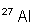Search:

# Learn to calculate with elementary particles- Number of protons = Z - Number of neutrons = A-Z - Number of electrons = Z-Q - Mass of the atom (in dalton) = A

1 atomic mass unit = 1 dalton = $1,66\cdot 10^{-27}$ kg

Examples:has 16 $p^+$ , 32-16 = 16 $n^0$, 16-(-2 ) = 18 $e^-$ and so its mass equals 32 dalton = $5,31\cdot 10^{-26}$ kghas 55 $p^+$ , 133-55 = 78 $n^0$, 55-(+1 ) = 54 $e^-$ and so its mass equals 133 dalton = $2,20\cdot 10^{-25}$ kghas 13 $p^+$ , 27-13 = 14 $n^0$, 13-0 = 13 $e^-$ and so its mass equals 27 dalton = $3,59\cdot 10^{-26}$ kg